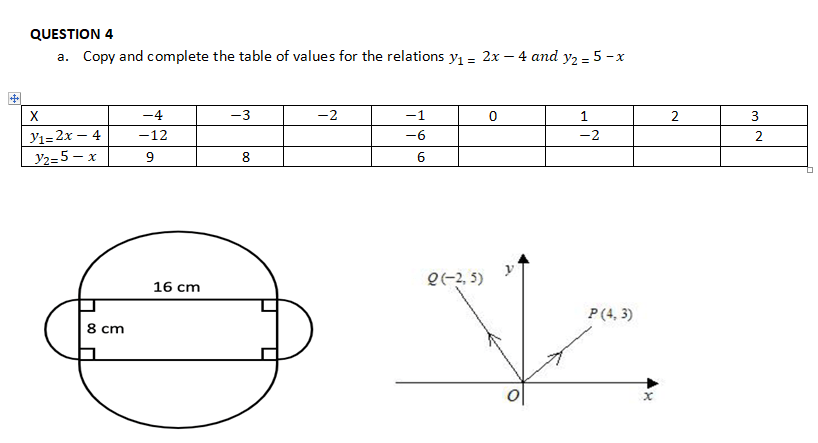# Likely Mathematics Questions for 2022 BECE CandidatesAll BECE candidates are to revise the following Likely Mathematics Questions for 2022 BECE Candidates. Each question should be attempted. Understand the requirements and show workings throughout the attempt.

## Likely Mathematics Questions for 2022 BECE Candidates

Answer only four questions from this section. All questions carry equal marks.
QUESTION 1
a. Solve for the value of x in the equation (8x-6)/4 =(16x-20)/16 + 46/8
b. Three coins are tossed once. Write down the set of possible outcomes. What is the probability of obtaining
iv. two heads and a tail
c. A closed cylinder has a radius of 4cm and height 9cm. Find its total surface area. [Take π=22/7]

Get the full set of questions and answers for Sections A and B here [Full Maths Questions and Answers]

Get other subjects:  Likely 2022 ICT BECE Questions for Candidates – Download

Get other subjects: Likely 2022 RME BECE Questions for (September Mock) for Candidates

Get Other Subjects: Over 2000 BECE Mock Questions, Likely Exam Questions And Answers (ALL SUBJECTS)

Get Other Subjects: Likely 2022 Pre Tech Skills BECE Questions for (Sept. Mock) for Candidates

Get other subjects:  Likely 2022 RME BECE Questions for (September Mock) for Candidates

QUESTION 2
a. The position vector of P is (4¦3) and the position vector of Q is ((-2)¦5)i. Find the vector 2(PQ) ⃗
ii. Calculate| (PQ ) ⃗| leaving your answer in the form √a

b. The average ages of 30 J.H.S 1 and 15 J.H.S 2 students in a school are 16 years and 19 years respectively. Find the average age of students of the two classes.
c. Find the truthset of (2x+3)/4 – (3x-2)/5 -(6x -5)/20 ≤ (-13)/5

QUESTION 3
a. The diagram below shows a playing field. Two opposite ends are semi-circles with diameter 8cm and 16cm. The straight sides are parallel.
Calculate, correct to the nearest whole numberi. The distance around the field
ii. The area of the field.[take π =3.142]

Likely Mathematics Questions for 2022 BECE Candidates

## QUESTION 4

a. Copy and complete the table of values for the relations y_(1 = ) 2x-4 and y_(2 = ) 5 –xb.i. Using a scale of 2cm to 1 unit on the x- axis and 2cm to 2 units on the y- axis, draw two perpendicular axes OX and OY on a graph sheet.
ii. On the same graph sheet, draw the graphs of the relations y_(1 =) 2x -4 and y_(2 =) 5-x
c. Find the coordinates of the point where y_(1 ) and y_(2 )meet

## QUESTION 5

a. The average of three numbers is 27. The second number is three times the first while the third is twice the first. Find the numbers
b. If P={ multiples of 2 less than 20}
Q= {multiple of 4 less than 17 }
R= {11<x≤16}
List the members of the following if Q and R are subsets of P
(i) P, Q and R
(ii)Q^(1 ) n R^1
(iii)(Q n 〖R)〗^1
c. Find the equation of the line passing through the points U(2,-5) and V(5,5).

Likely Mathematics Questions for 2022 BECE Candidates. Get the full set of questions and answers for Sections A and B here [Full Maths Questions and Answers]

## QUESTION 6

a. If  23n= 1111 base 2 find n.
b. A shoe distributing company accepts GH¢130.40 instead of the catalogue price of GH¢140.40 per pair of shoes.

The company does this to encourage prompt payment. What is the cash discount percent?
c. If P= m/R^2 +k, make R the subject of the relation.